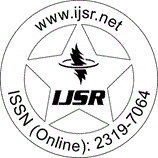International Journal of Science and Research (IJSR)
Call for Papers | Fully Refereed | Open Access | Double Blind Peer Reviewed

Downloads: 119 | Views: 175 | Weekly Hits: ⮙1 | Monthly Hits: ⮙1

Research Paper | Aerospace Engineering | India | Volume 9 Issue 5, May 2020

# Laminar Flow Prediction over a Rotating Disc using Bezier Curves

Athira Manikandan  | Pravin Kumar Kamanat 

Abstract: An analysis of fluid dynamic characteristics of the interaction of a laminar flow with a circular disc rotating at uniform angular velocity, is presented. The study emphasizes on a novel method of using parametric Bezier curves for the representation of the solution to the flow problem, which due to the assumptions involved simplifies into exact solutions of three-dimensional flow, i. e. , three dimensional Navier Stokes equations near the rotating disk. A MATLAB code has been written to implement the method, whose results are compared with the numerical solution given by the conventional Boundary value problem solver. It is predicted that due to the precision of the results obtained, the novel method is an appropriate means to solve the systems of nonlinear equations. It can have comprehensive application in complex mathematical and engineering problems.

Keywords: Bezier Curves, Navier-Stokes Equations, Boundary Value problem

Edition: Volume 9 Issue 5, May 2020,

Pages: 1767 - 1776